#DAY 14
0
Modern Web

## Day14－D3 的 Force 原力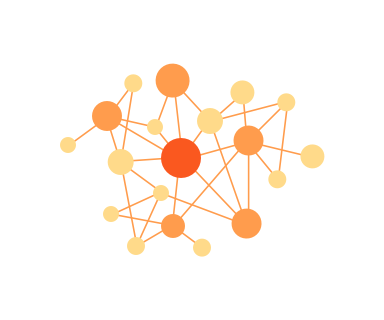## 引力與斥力

• index ⇒ 第幾個的元素
• name ⇒ 綁定的資料
• vx, vy ⇒ 速度
• x, y ⇒ 位置
• (fx, fy) ⇒ 固定的位置，預設是沒有的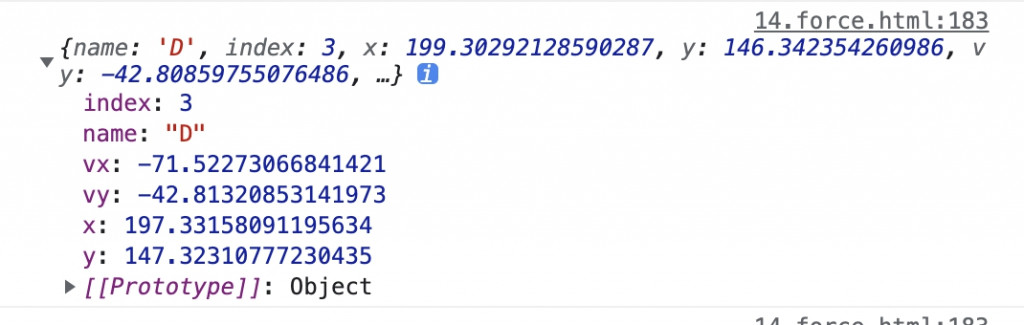## 五種作用力

• 中心力 ⇒ `d3.forceCenter`

中心力作用於所有的節點而不是單獨的節點。它可以把所有節點的中心一致朝指定的位置平移，而且這種移動不會修改速度也不會影響節點間的相對位置。

forceCenter 旗下有一些API 可以進行相關設定：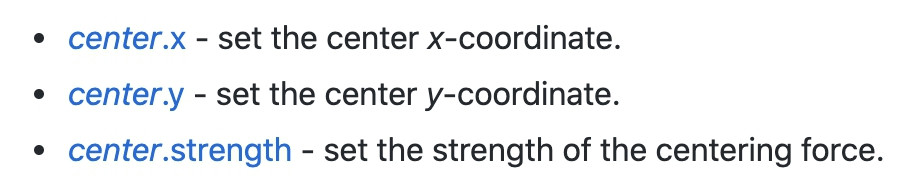• 碰撞力 ⇒ `d3.forceCollide`

節點之間互相排斥的斥力（相互碰撞排斥），這個斥力會阻止節點相互重疊，可以使用 .strength( ) 這個API去設定斥力的強度

forceCollide 旗下有一些API 可以進行相關設定：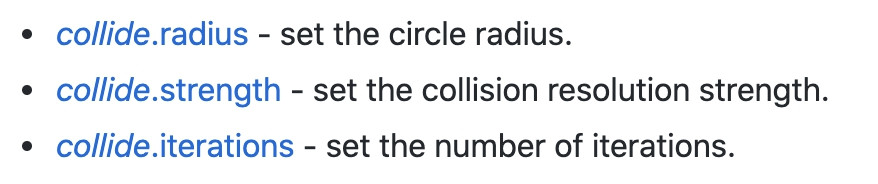• 連結力 ⇒ `d3.forceLink`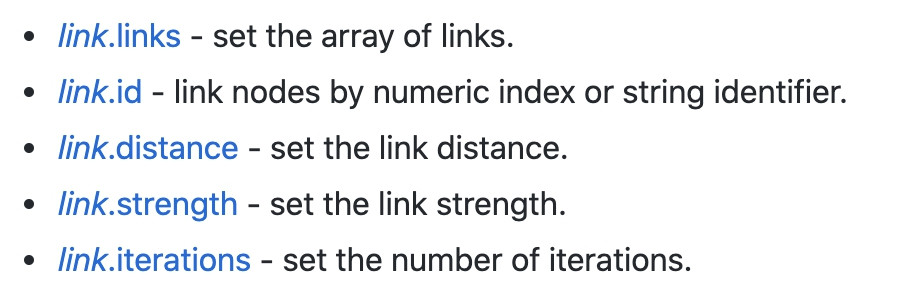• 電荷力 ⇒ `d3.forceManyBody`

模擬所有節點間的相互作用力，如果設定是正值，節點間就會相互吸引；如果設定是負值，節點間就會相互排斥。這樣可以用來模擬電荷吸引力，而力的大小也和節點間的距離有關。

forceManyBody 旗下有一些API 可以進行相關設定：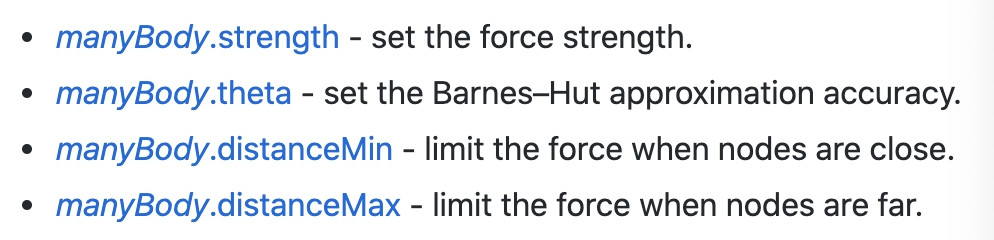• 定位力 ⇒ `d3.forceX``d3.forceY``d3.forceRadial`

定位力可以把節點沿著指定的維度推向一個指定位置，這些位置又可以透過三個不同的API去設定

• d3.forceX：在 X軸方向推或者拉所有的節點

• d3.forceY：在 Y軸 方向推或者拉所有的節點

這三個方法旗下也有一些 API 可以進行相關設定：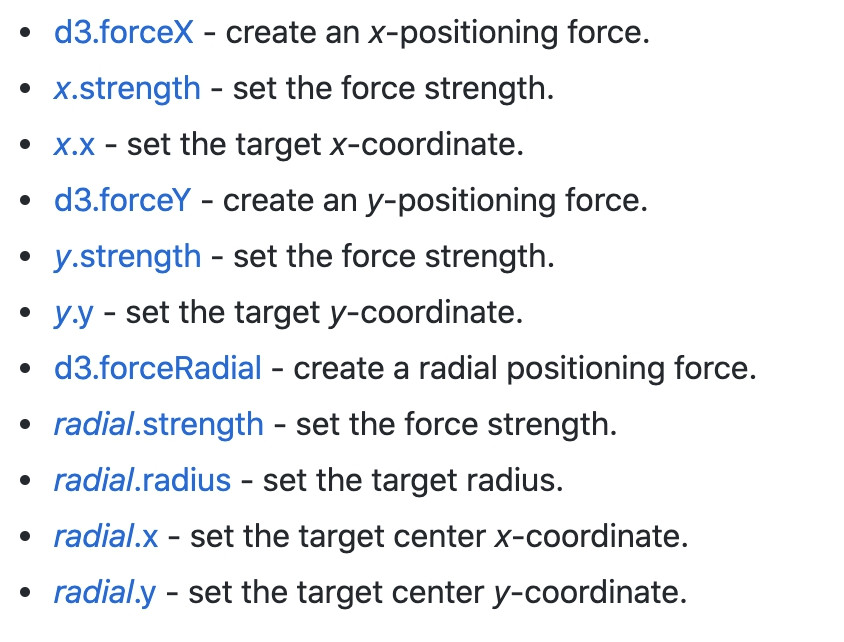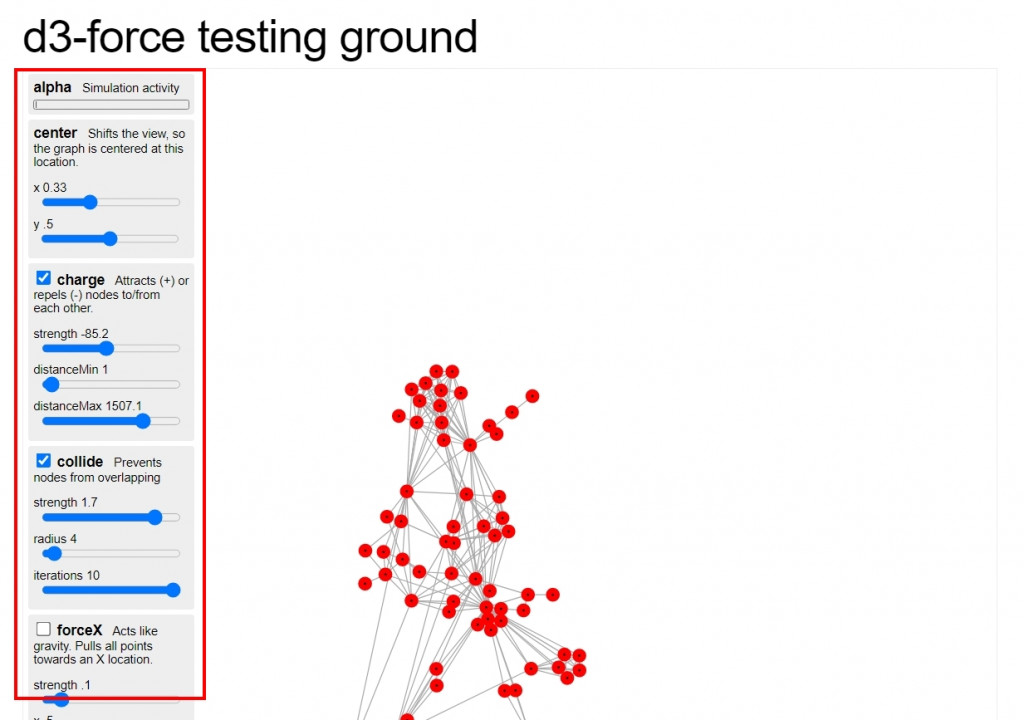### `d3.forceSimulation( )`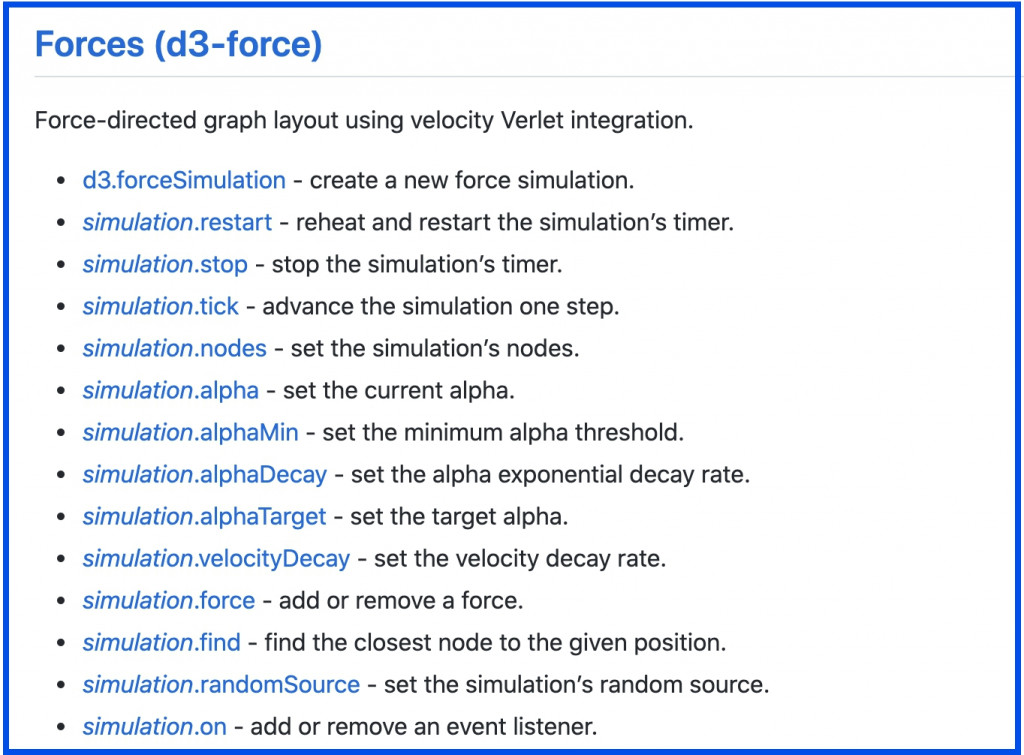• `simulation.restart``simulation.stop``simulation.tick`

這幾個方法是來控制模擬器重新執行、停止、停止後手動前進

• `simulation.nodes`

將力模擬圖建立的元素與資料綁定

• `simulation.alpha``simulation.alphaMin``simulation.alphaDecay`

前面有提過，力模擬圖的節點們向中心聚攏的收斂方式是使用 alpha 的參數去控制，這幾個 API 就是用來控制 alpha 的值。在力模擬圖一建立並開始執行時，alpha 值設定為1，並在經過 300次迭代 (iterations) 之後衰減為0，這時候就會看到整個力模擬圖呈現靜止的狀態。如果希望力模擬圖永遠不靜止的話，可以把 alpha 的參數設定為0

— simulation.alphaMin：alpha 小於 alphaMin 設定的值時，力模擬器就會停止運作

• `simulation.velocityDecay`

設定摩擦力的係數，數值1是無摩擦力的狀態(粒子運動很快)，數值0則會讓所有粒子失去速度(凍結靜止)，

• `simulation.force(name[,force])`

建立好一個力模擬器後，我們可以使用 simulation.force() 把前面提到的五種不同作用力當成參數添加到力模擬器中。第一個參數name可以任意自訂想取的名稱，第二個參數則填入要使用的作用力。舉幾個常見的例子來說：

``````const simulation = d3.forceSimulation(nodes)
// 設定節點連結的引力
// 在 y軸方向上施加一個力
.force('y', d3.forceY().strength(0.025))
// 節點間的電荷力
.force('charge', d3.forceManyBody())
// 節點間的斥力
//中心點的引力
.force('center', d3.forceCenter(width / 2, height / 2))
``````
• `simulation.on(事件, 方法)`

啟動模擬器的事件監聽，並設定之後的callback function。力模擬器的事件監聽有兩種：

tick ⇒ 內部定時器每次運行 tick 之後

end ⇒ 當alpha < alphaMin，因此定時器停止之後

### 範例一：力圖

``````// html
<svg class="forceElement" style="width:500; height:300"></svg>

// js
const data = [{name:'A'},{name:'B'},{name:'C'},{name:'D'},{name:'E'},{name:'F'},{name:'G'}]
``````

``````const dots =  d3.select('.forceElement')
.append('g')
.selectAll('circle')
.data(data)
.enter()
.append('circle')
.attr('cx', 250)
.attr('cy', 150)
.attr('r', 15)
.style('fill', 'green')
.style('opacity', 0.4)
``````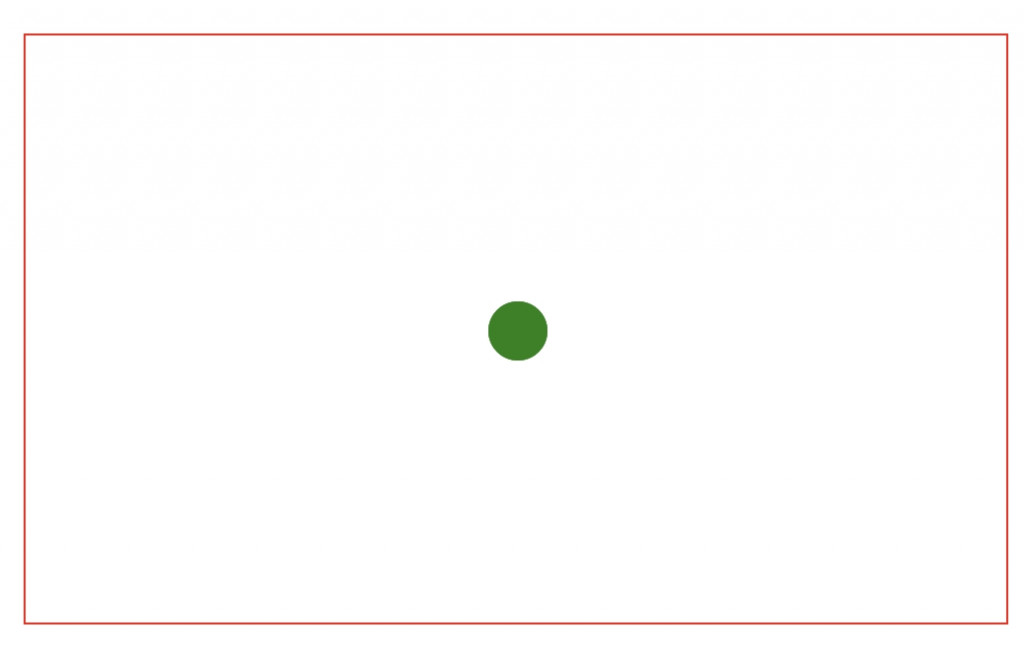``````// 設定力模擬
const simulation = d3.forceSimulation()
.velocityDecay(0.2) // 設定摩擦係數
.force("x", d3.forceX()) // 設定Ｘ軸平移位置
.force("y", d3.forceY()) // 設定Ｙ軸移動位置
// 設定中心點位置
.force("center", d3.forceCenter().x(250).y(150))
// 設定節點間電荷力
.force("charge", d3.forceManyBody().strength(1))
// 設定節點間彼此的互斥力
``````

``````// 將力模擬器的節點綁定資料，設定ticks開始時節點的動作
simulation.nodes(data)
.on("tick", function(d){
dots.attr("cx", d => d.x)
.attr("cy", d => d.y)
});
``````### 範例二：力圖-資料分組

``````// html
<svg class="forceGroup" style="width:500; height:300"></svg>

//js
const data = [
{ "name": "A", "group": 150 },
{ "name": "B", "group": 150 },
{ "name": "C", "group": 150 },
{ "name": "D", "group": 150 },
{ "name": "E", "group": 150 },
{ "name": "F", "group": 150 },
{ "name": "G", "group": 250 },
{ "name": "H", "group": 250 },
{ "name": "I", "group": 250 },
{ "name": "J", "group": 250 },
{ "name": "K", "group": 250 },
{ "name": "L", "group": 350 },
{ "name": "M", "group": 350 },
{ "name": "N", "group": 350 },
{ "name": "O", "group": 350 }
]

// 設定顏色
const colorScale = d3.scaleOrdinal()
.domain([150, 250, 350])
.range(["red", "blue", "orange"])

// 建立圓點，全都位於正中央
const node = d3.select('.forceGroup')
.append('g')
.selectAll("circle")
.data(data)
.enter()
.append('circle')
.attr('r', 20)
.attr('cx', 250)
.attr('cy', 150)
.style('fill', d=>colorScale(d.group))
.style('opacity', '0.6')
``````

``````// 設定力模擬器
const simulation = d3.forceSimulation()
.force("x", d3.forceX().strength(0.5).x(d => d.group))
.force("y", d3.forceY().strength(0.1).y( 150 ))
.force("center", d3.forceCenter().x(250).y(150))
.force("charge", d3.forceManyBody().strength(1))
``````

``````// 將力模擬器的節點綁定資料
simulation.nodes(data)
.on("tick", function(d){
node.attr("cx", d => d.x)
.attr("cy", d => d.y)
});
``````### 範例三：力圖-資料連結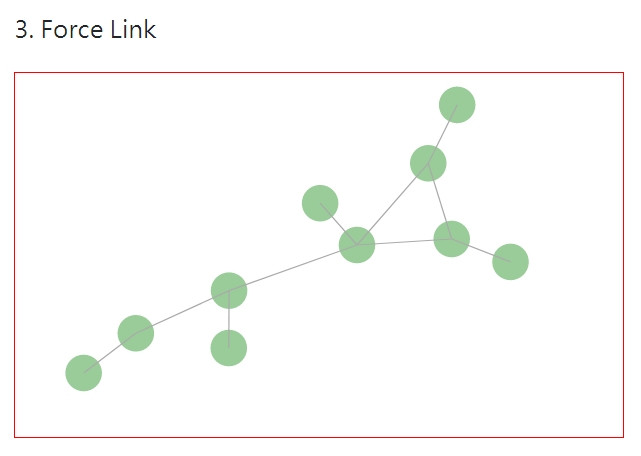• source ⇒ 目前的點
• target ⇒ 要串接的點

``````// html

// js
const data = {
"nodes": [
{"id": 1, "name": "A"},
{"id": 2, "name": "B"},
{"id": 3, "name": "C"},
{"id": 4, "name": "D"},
{"id": 5, "name": "E"},
{"id": 6, "name": "F"},
{"id": 7, "name": "G"},
{"id": 8, "name": "H"},
{"id": 9, "name": "I"},
{"id": 10, "name": "J"}
],
{"source": 1, "target": 2},
{"source": 1, "target": 3},
{"source": 1, "target": 6},
{"source": 2, "target": 3},
{"source": 2,"target": 7},
{"source": 3,"target": 4},
{"source": 8,"target": 3},
{"source": 4,"target": 5},
{"source": 4,"target": 9},
{"source": 5,"target": 10}
]
}
``````

``````const dots =  d3.select('.forceLink')
.append('g')
.selectAll('circle')
.data(data.nodes)
.enter()
.append('circle')
.attr('r', 15)
.style('fill', 'green')
.style('opacity', 0.4)

.selectAll("line")
.join("line")
.style("stroke", "#aaa")
``````

``````  // 設定力模擬器
const simulation = d3.forceSimulation(data.nodes)
.force("charge", d3.forceManyBody().strength(-300))
.force("center", d3.forceCenter(250, 150))
.on('tick', ticked)

// 設定 ticked 方法
function ticked(d){
.attr("x1", function(d) { return d.source.x; })
.attr("y1", function(d) { return d.source.y; })
.attr("x2", function(d) { return d.target.x; })
.attr("y2", function(d) { return d.target.y; });

dots.attr("cx", d=> d.x)
.attr("cy", d => d.y);
}
``````

### 範例四：力圖-結合hover``````// html
<div class="hoverWrap position-relative">
<svg class="forceHover" style="width:500; height:300"></svg>
</div>

// js
const data = [{'r': 3}, {'r':34}, {'r': 23}, {'r': 33},
{'r': 13},{'r': 22},{'r': 43},{'r': 17},{'r': 38}]

const dots = d3.select('.forceHover')
.selectAll('circle')
.data(data)
.enter()
.append('circle')
.attr('r', d => d.r)
.attr('fill', 'blue')
.attr('opacity', 0.3)
// 設定力模擬器
const simulation = d3.forceSimulation()
.force("center", d3.forceCenter().x(250).y(150))
.force("charge", d3.forceManyBody().strength(.3))
//綁定資料
simulation.nodes(data)
.on('tick', function(d){
dots.attr('cx', d=>d.x)
.attr('cy', d=>d.y)
})
``````

``````// 建立tooltips
const tooltips = d3.select(".hoverWrap")
.append("div")
.style("opacity", 0)
.style('position', 'absolute')
.attr("class", "tooltip")
.style("background-color", "white")
.style("border", "solid")
.style("border-width", "2px")
// hover
dots.on('mouseover', mouseover)
.on('mouseleave', mouseleave)
``````

``````function mouseover(event, d){
console.log(d)
d3.select(this)
.attr('stroke', 'black')
.attr('stroke-width', '3px')
.attr('opacity', 0.7)
.style('cursor', 'pointer')

let pt = d3.pointer(event, this)
tooltips.style('opacity', 1)
.style('left', pt+ 10 +'px')
.style('top', pt+'px')
.html(`半徑：\${d.r}`)
}

function mouseleave(event, d){
d3.select(this)
.attr('stroke', 'none')
.attr('stroke-width', '0')
.attr('opacity', 0.3)

tooltips.style('opacity', 0)
}
``````

### 範例五：力圖-結合drag``````// 資料
const data = [{name:'A'},{name:'B'},{name:'C'},{name:'D'},{name:'E'},{name:'F'},{name:'G'}]

// 建立原點，目前全部都在同個位置
const dots = d3.select('.forceDrag')
.append('g')
.selectAll('circle')
.data(data)
.enter()
.append('circle')
.attr('r', 25)
.attr('cx', 50)
.attr('cy', 50)
.style("fill", "#19d3a2")
.style("fill-opacity", 0.3)
.attr("stroke", "#b3a2c8")
.style("stroke-width", 4)
.style('cursor', 'pointer')

// 建立力模擬圖
const simulation = d3.forceSimulation()
.force("center", d3.forceCenter().x(200).y(150))
.force("charge", d3.forceManyBody().strength(1))

simulation.nodes(data)
.on("tick", function(d){
dots.attr("cx", d => d.x)
.attr("cy", d => d.y)
});
``````

``````// 拖曳開始
function dragstarted(event, d) {
// console.log(d)
d3.select(this)
.style('fill-opacity', 0.6)
d.fx = d.x;
d.fy = d.y;
simulation.alphaTarget(.03).restart();
}
// 拖曳期間
function dragged(event, d) {
d.fx = event.x;
d.fy = event.y;
}
// 拖曳結束
function dragended(event, d) {
simulation.alphaTarget(.03);
d3.select(this)
.style('fill-opacity', 0.3)
d.fx = null;
d.fy = null;
}

// 綁定拖曳事件
dots.call(d3.drag()
.on("start", dragstarted)
.on("drag", dragged)
.on("end", dragended));
``````

``````// html

// js
const data = {
"nodes": [
{"id": 1, "name": "A"},
{"id": 2, "name": "B"},
{"id": 3, "name": "C"},
{"id": 4, "name": "D"},
{"id": 5, "name": "E"},
{"id": 6, "name": "F"},
{"id": 7, "name": "G"},
{"id": 8, "name": "H"},
{"id": 9, "name": "I"},
{"id": 10, "name": "J"}
],
{"source": 1, "target": 2},
{"source": 1, "target": 3},
{"source": 1, "target": 6},
{"source": 2, "target": 3},
{"source": 2,"target": 7},
{"source": 3,"target": 4},
{"source": 8,"target": 3},
{"source": 4,"target": 5},
{"source": 4,"target": 9},
{"source": 5,"target": 10}
]
}

.append('g')
.selectAll('circle')
.data(data.nodes)
.enter()
.append('circle')
.attr('r', 15)
.style('fill', 'green')
.style('opacity', 0.6)
.style('cursor', 'pointer')

.selectAll("line")
.join("line")
.style("stroke", "#aaa")

// 設定力模擬器
const simulation = d3.forceSimulation(data.nodes)
.force("charge", d3.forceManyBody().strength(-300))
.force("center", d3.forceCenter(250, 150))
.on('tick', ticked)

// 綁定節點
function ticked(d){
.attr("x1", function(d) { return d.source.x; })
.attr("y1", function(d) { return d.source.y; })
.attr("x2", function(d) { return d.target.x; })
.attr("y2", function(d) { return d.target.y; });

dots.attr("cx", d=> d.x)
.attr("cy", d => d.y);
}
``````

``````// 綁定拖曳事件
dots.call(d3.drag()
.on("start", dragstarted)
.on("drag", dragged)
.on("end", dragended));

function dragstarted(event, d){
d3.select(this)
.style('fill', 'pink')
d.fx = d.x;
d.fy = d.y;
simulation.alphaTarget(.03).restart(); // 停止後需要重新開始
}

function dragged(event, d){
d.fx = event.x;
d.fy = event.y;
}

function dragended(event, d){
d3.select(this)
.style('fill', 'green')
.style('opacity', 0.6)
d.fx = null
d.fy = null
}
``````